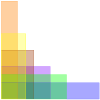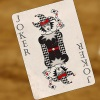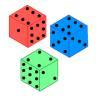Search by Topic

Resources tagged with Curious similar to Arithmagons:

Filter by: Content type:
Age range:
Challenge level:

There are 68 results

Broad Topics > Habits of Mind > CuriousArithmagons

Age 14 to 16 Challenge Level:

Can you find the values at the vertices when you know the values on the edges?Multiplication Arithmagons

Age 14 to 16 Challenge Level:

Can you find the values at the vertices when you know the values on the edges of these multiplication arithmagons?Pick's Theorem

Age 14 to 16 Challenge Level:

Polygons drawn on square dotty paper have dots on their perimeter (p) and often internal (i) ones as well. Find a relationship between p, i and the area of the polygons.What's Possible?

Age 14 to 16 Challenge Level:

Many numbers can be expressed as the difference of two perfect squares. What do you notice about the numbers you CANNOT make?Can They Be Equal?

Age 11 to 14 Challenge Level:

Can you find rectangles where the value of the area is the same as the value of the perimeter?Pair Products

Age 14 to 16 Challenge Level:

Choose four consecutive whole numbers. Multiply the first and last numbers together. Multiply the middle pair together. What do you notice?Beelines

Age 14 to 16 Challenge Level:

Is there a relationship between the coordinates of the endpoints of a line and the number of grid squares it crosses?How Much Can We Spend?

Age 11 to 14 Challenge Level:

A country has decided to have just two different coins, 3z and 5z coins. Which totals can be made? Is there a largest total that cannot be made? How do you know?Searching for Mean(ing)

Age 11 to 14 Challenge Level:

Imagine you have a large supply of 3kg and 8kg weights. How many of each weight would you need for the average (mean) of the weights to be 6kg? What other averages could you have?Cuboid Challenge

Age 11 to 16 Challenge Level:

What's the largest volume of box you can make from a square of paper?What Numbers Can We Make Now?

Age 11 to 14 Challenge Level:

Imagine we have four bags containing numbers from a sequence. What numbers can we make now?Summing Consecutive Numbers

Age 11 to 14 Challenge Level:

15 = 7 + 8 and 10 = 1 + 2 + 3 + 4. Can you say which numbers can be expressed as the sum of two or more consecutive integers?How Old Am I?

Age 14 to 16 Challenge Level:

In 15 years' time my age will be the square of my age 15 years ago. Can you work out my age, and when I had other special birthdays?Litov's Mean Value Theorem

Age 11 to 14 Challenge Level:

Start with two numbers and generate a sequence where the next number is the mean of the last two numbers...Think of Two Numbers

Age 11 to 14 Challenge Level:

Think of two whole numbers under 10, and follow the steps. I can work out both your numbers very quickly. How?Largest Product

Age 11 to 14 Challenge Level:

Which set of numbers that add to 10 have the largest product?Age 11 to 14 Challenge Level:

Think of a number and follow my instructions. Tell me your answer, and I'll tell you what you started with! Can you explain how I know?What Numbers Can We Make?

Age 11 to 14 Challenge Level:

Imagine we have four bags containing a large number of 1s, 4s, 7s and 10s. What numbers can we make?A Little Light Thinking

Age 14 to 16 Challenge Level:

Here is a machine with four coloured lights. Can you make two lights switch on at once? Three lights? All four lights?Special Numbers

Age 11 to 14 Challenge Level:

My two digit number is special because adding the sum of its digits to the product of its digits gives me my original number. What could my number be?More Number Pyramids

Age 11 to 14 Challenge Level:

When number pyramids have a sequence on the bottom layer, some interesting patterns emerge...Number Pyramids

Age 11 to 14 Challenge Level:

Try entering different sets of numbers in the number pyramids. How does the total at the top change?On the Edge

Age 11 to 14 Challenge Level:

If you move the tiles around, can you make squares with different coloured edges?Perimeter Possibilities

Age 11 to 14 Challenge Level:

I'm thinking of a rectangle with an area of 24. What could its perimeter be?Unequal Averages

Age 11 to 14 Challenge Level:

Play around with sets of five numbers and see what you can discover about different types of average...Curvy Areas

Age 14 to 16 Challenge Level:

Have a go at creating these images based on circles. What do you notice about the areas of the different sections?Blue and White

Age 11 to 14 Challenge Level:

Identical squares of side one unit contain some circles shaded blue. In which of the four examples is the shaded area greatest?Sending a Parcel

Age 11 to 14 Challenge Level:

What is the greatest volume you can get for a rectangular (cuboid) parcel if the maximum combined length and girth are 2 metres?Marbles in a Box

Age 11 to 16 Challenge Level:

How many winning lines can you make in a three-dimensional version of noughts and crosses?Cola Can

Age 11 to 14 Challenge Level:

An aluminium can contains 330 ml of cola. If the can's diameter is 6 cm what is the can's height?Estimating Time

Age 11 to 14 Challenge Level:

How well can you estimate 10 seconds? Investigate with our timing tool.Satisfying Statements

Age 11 to 14 Challenge Level:

Can you find any two-digit numbers that satisfy all of these statements?Which Solids Can We Make?

Age 11 to 14 Challenge Level:

Interior angles can help us to work out which polygons will tessellate. Can we use similar ideas to predict which polygons combine to create semi-regular solids?Statement Snap

Age 7 to 14 Challenge Level:

You'll need to know your number properties to win a game of Statement Snap...Non-transitive Dice

Age 11 to 14 Challenge Level:

Alison and Charlie are playing a game. Charlie wants to go first so Alison lets him. Was that such a good idea?Take Three from Five

Age 14 to 16 Challenge Level:

Caroline and James pick sets of five numbers. Charlie chooses three of them that add together to make a multiple of three. Can they stop him?A Chance to Win?

Age 11 to 14 Challenge Level:

Imagine you were given the chance to win some money... and imagine you had nothing to lose...Reversals

Age 11 to 14 Challenge Level:

Where should you start, if you want to finish back where you started?What's it Worth?

Age 11 to 16 Challenge Level:

There are lots of different methods to find out what the shapes are worth - how many can you find?Partly Painted Cube

Age 14 to 16 Challenge Level:

Jo made a cube from some smaller cubes, painted some of the faces of the large cube, and then took it apart again. 45 small cubes had no paint on them at all. How many small cubes did Jo use?Elevenses

Age 11 to 14 Challenge Level:

How many pairs of numbers can you find that add up to a multiple of 11? Do you notice anything interesting about your results?Square Coordinates

Age 11 to 14 Challenge Level:

A tilted square is a square with no horizontal sides. Can you devise a general instruction for the construction of a square when you are given just one of its sides?Opposite Vertices

Age 11 to 14 Challenge Level:

Can you recreate squares and rhombuses if you are only given a side or a diagonal?Right Angles

Age 11 to 14 Challenge Level:

Can you make a right-angled triangle on this peg-board by joining up three points round the edge?Semi-regular Tessellations

Age 11 to 16 Challenge Level:

Semi-regular tessellations combine two or more different regular polygons to fill the plane. Can you find all the semi-regular tessellations?Nice or Nasty

Age 7 to 14 Challenge Level:

There are nasty versions of this dice game but we'll start with the nice ones...Shifting Times Tables

Age 11 to 14 Challenge Level:

Can you find a way to identify times tables after they have been shifted up or down?Cosy Corner

Age 11 to 14 Challenge Level:

Six balls of various colours are randomly shaken into a trianglular arrangement. What is the probability of having at least one red in the corner?Two's Company

Age 11 to 14 Challenge Level:

7 balls are shaken in a container. You win if the two blue balls touch. What is the probability of winning?Who's the Best?

Age 11 to 14 Challenge Level:

Which countries have the most naturally athletic populations?